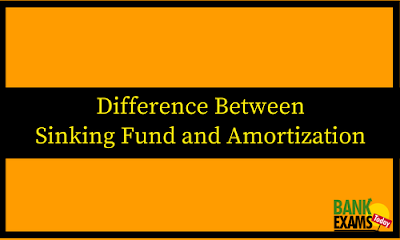New Student User - Use Code HELLO

# Difference Between Sinking Fund and Amortization## Introduction

For investors, there are a number of options and sources from where to arrange a fund for the investment. Funds can either borrowed or use a fund which was kept aside for future use. A sinking fund is one type of investment which was kept aside for future expectancy on the contrary amortization is a debt instrument like a loan or a mortgage which is paid in installments. Both have a notable difference between the characteristics of time, interest calculation method etc.

## Sinking Fund

• A sinking fund is managed by arranging aside fund over a span of time to meet prospective future expenses.
• A fund will be kept in an account which earns compound interest over a period of time. It means an interest occurred on the original sum is added to it and this process goes on. It is also called an interest on the internet.

### For example:

A business entity has made a deposit of Rs 1000 in the initial at the rate of interest 12 percent, so the interest on deposit is Rs 120 per month and for the second month an interest of Rs 120 is RS 1000 which is same as the first month. The same process continues until the maturity ends. There is a formula by which an investor is able to know the future value his fund at the time of maturity.

FV= PV (1+r) n    Where,
FV= Future Value of the fund (at its maturity)
PV= Present Value (the amount that should be invested today)
r = Rate of return
n = Number of time periods

## Amortization

• Amortization is a periodic payment of a debt like a loan or a mortgage.
• Each periodic installment will include a principal amount as well as interest. Repayment installments are divided into equal amounts for the duration of the loan.
• Amortization is the arrangement of a lump sum cash flow into many periodic installments over a span of time, which is also called amortization agenda.
• Amortization is a word used to account for the reduction of the value of capital assets over a span. This is similar to the noncash expenditure like a devaluation which is done only for the intangible assets like Patents, copyrights, and trademarks.

### For Example

A business entity has made a deposit of Rs 1000 in the initial at the rate of interest 12 percent, so the interest on deposit is Rs 120 per month.and for the second month an interest of Rs 120 is added to the principal amount that is RS 1000. So, now the initial amount for the second month is Rs 1120 and the interest calculated on this amount. The same process continues until the maturity ends.

## Difference Between Sinking Fund and Amortization

### Meaning:

• A sinking fund is managed by arranging aside fund over a span of time to meet prospective future expenses.
• Amortization is the arrangement of a lump sum cash flow into many periodic installments over a span of time.

### Interest amount:

• In sinking fund, an interest amount is received from the borrower.
• In amortization, an interest amount is paid to the lender.

• In sinking fund, you can only purchase with cash in hand and this is possible when you had kept aside a fund for future.
• In amortization, one will get a loan to purchase anything in the initial and pay it with interest at the end of maturity.

### Factors

• In sinking fund, one knows the fund one want at the end of the period. So, one will calculate the amount one has kept aside each installemts to get that amount.
• In amortization, one knows a borrowed amount and the lender calculates the amount of each periodic installment for the borrower and the borrower knows the amount to pay until amortization period ends.